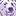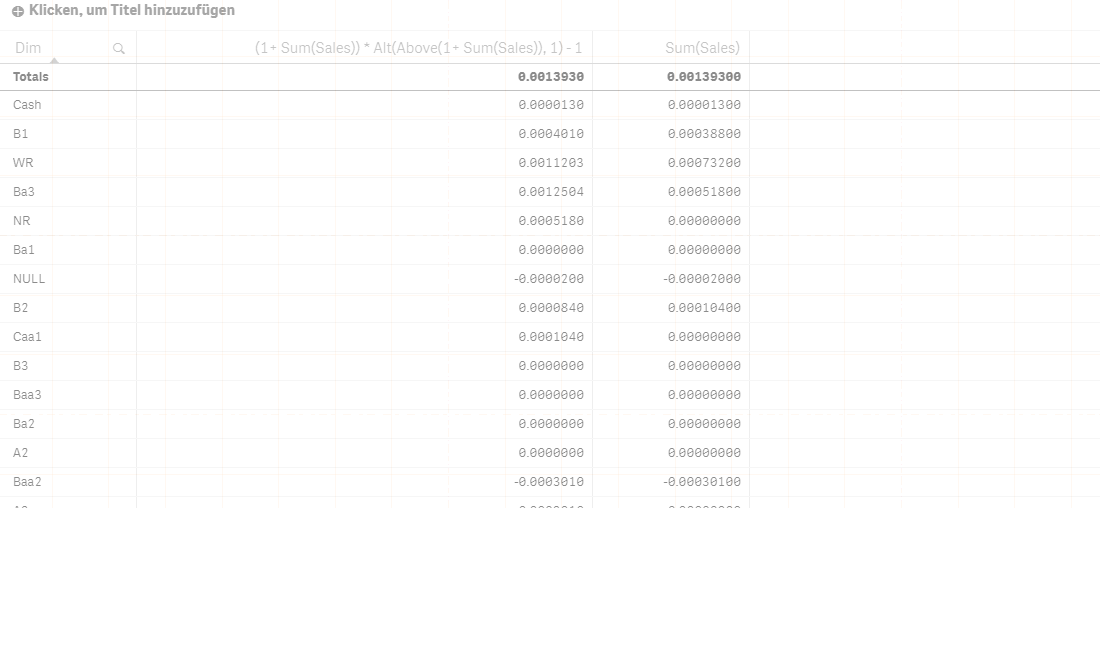App Development

Announcements
QLIKWORLD LIVE! MAY 16 - 19TH, EARLY BIRD DISCOUNTS! REGISTER TODAY
cancel
Showing results for
Did you mean:Contributor

I want to perform Multiply(Sales) instead of Sum(Sales). How can i do it in qlik sense.

How can i achieve multiply(sales) in qlik sense

1 Solution

Accepted SolutionsMaster II

Hi

check this out and test the data and tell me  if it works.

hopefully this is what you want.

edit: sorry i forget to -1 . this is just the multiplication. you need to minus 1 to get the result

regards

Learning never stops.
22 RepliesContributor
Author

More precise question..

Want to calculate something like this where T1,T2...Tn are the values from 'Timekey' dimension selected by the user

((1+Sum({<Timekey={'T1'}>}Sales)) * (1+Sum({<Timekey={'T2'}>}Sales)) * ....) - 1

How to achieve this ??Partner

Hello Sayyam,

maybe something like this will work?

(1+ Sum(Sales)) * (1+ Above(Sum(Sales))

MathiasContributor
Author

Thanks for replying Mathias , but how do i dynamically assign timekeys to my calculation.If you can look at sample qvf i have attached then you might be able to suggest some solution.Partner

I guess I don't understand your issue because I took a look at your qvf and still think my formula is correct.

Why would you want to assign timekeys to your calculation? If the user selects one or more timekeys Qlik Sense will only calculate the sales of the current selection.MathiasContributor
Author

Hi Mathias thanks again,

Sorry I am new to qlik so i might be confused, suppose i select 4 timekeys ,will this formula calculate 1+sum(Sales) seperately for each timekey and then multiply all those values ?Partner

Yes it will. Suppose you have a table like:

TimeKey         Sales

01/03/2017      100

01/03/2017      200

02/03/2017      300

03/03/2017      400

and an expression Sum(Sales) in a text object.

If you select 01/03/2017 the expression will result in 300.

If you select 01/03/2017 and 02/03/2017 the expression will result in 600.

If you select only 03/03/2017 the expression will result in 400.

I strongly recommend reading up on the basics of QlikView / Qlik Sense! (maybe try https://www.qlik.com/us/products/qlikview/getting-started)

MathiasContributor
Author

Hi Mathias

if i select 01/03/2017 and 02/03/2017 i dont want to simply add to get 600 i want (1+100+200) * (1+300)-1=90600

you get my point right,for 100+200 for 01/03/2017 and 300 for 02/03/2017 and then multiply the values.Partner

I was just giving a simplified example of how the Qlik selection logic works (using the table i posted as data and the expression I posted as a measure) because you said you are not familiar with Qlik Sense.

Did you try the expression I posted earlier?Master II

Hi

check this out and test the data and tell me  if it works.

hopefully this is what you want.

edit: sorry i forget to -1 . this is just the multiplication. you need to minus 1 to get the result

regards